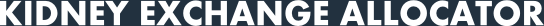## Optimality Criteria

### Introduction

In this section we describe exactly what it means when we say that the output from the algorithm is optimal. This definition of optimal dictates exactly what is output when the `operation` parameter in the various API calls is set to `optimal`. We also describe the constraints on the set of exchanges when the parameters `maxcard` and `pairs` are used.

### Optimal

When the operation parameter to the various API calls is set to optimal we return a set of exchanges that are derived from a set of constraints as defined below:

1. the number of effective pairwise exchanges is maximised; [*]
2. subject to (1), the total number of transplants is maximised;
3. subject to (1)-(2), the number of altruistic donors involved in a (pairwise) exchange is maximised;
4. subject to (1)-(3), the number of 3-way exchanges is minimised;
5. subject to (1)-(4), the number of back-arcs in the 3-way exchanges is maximised;
6. subject to (1)-(5), the overall weight is maximised.

[*] And thus equal to the number of pairwise exchanges in a maximum cardinality solution consisting of only pairwise exchanges.

### Maxcard

When the operation parameter is set to `maxcard` we relax somewhat the set of constraints that are applied. Running the algorithms with this setting returns us the largest possible matching in terms of size, i.e. it is not constrainted by maximising the overall number of effective pairwise exchanges, or by altruistic donors. The exact set of criteria are shown below:

1. the total number of transplants is maximised;
2. subject to (1), the overall weight is maximised.

### Pairs

The `pairs` operation returns the set of pairwise exchanges such that:

1. the total number of transplants is maximised;
2. subject to (1), the overall weight is maximised.
v1.0.0